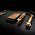Using the midpoint formula to solve elasticity questions in economics - FreeEconHelp.com, Learning Economics... Solved!

## 8/17/11

Most economics classes will require you to use the midpoint formula in order to solve elasticity questions.  They require this because a percent change in a given problem could be different depending on whether the price is increasing, or falling.  Check out the example below for a price change from \$5 to \$10:

If the price increases to \$10, then we have (\$10-\$5)/\$5, which gives us \$5/\$5, or 100%

However if the price decreases we have (\$5-\$10)/\$10, which gives us -\$5/\$10, or -50%.

So depending on whether it is a price increase or decrease, then we will see different percentage
values.  But if we use the midpoint formula, this won’t be a problem.  Let us look at our example above again.

Using the midpoint formula, we have to take the average of the beginning and ending price, this gives us \$7.50 or (\$5+\$10)/2.  So for a price increase we get:

(\$10-\$5)/\$7.50 or \$5/\$7.50 which gives us a percent change of 66.67%.

We can then do the same analysis for a price decrease:

(\$5-\$10)/\$7.50 or -\$5/\$7.50 which gives us the same percent change of 66.67%.

So the question at hand, is to find the price elasticity of demand for candy which the price increases from \$0.85 to \$0.95, and consumption decreases from 450,000 unit to 350,000 per month.
We set up the equation in the following manner, ending price minus initial price divided by average price (using the midpoint formula), divided by ending quantity minus initial quantity divided by average quantity (discussion of elasticity problems and discussion of elastic vs inelastic).  This looks like:

So our answer is -4/9 or -.44444.  The answer is negative because as the price goes up, we consume less of the good (which follows the law of demand).  You can see in the equations that the use of the midpoint formula simply gave us the average between the initial and ending values, which enters into the denominator for both the price and quantity change.

1.I think your formula is upside down homie...

2.pretty sure the right answer is -2.25 ;-P

3.This is wrong. Please correct your formula to have the % change in price in the denominator and the % change in quantity demanded in the numerator. The correct answer, as stated by other commenters, is -2.25. Additionally, since elasticity of demand is always negative the negative sign is generally dropped for simplicity.

4.Your formula is upside down %Change in QD / %Change in Price.